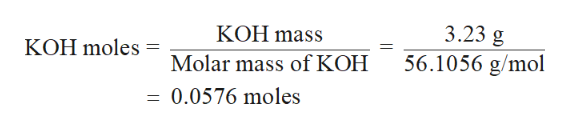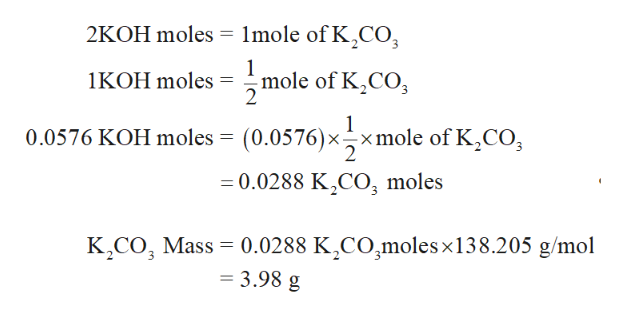# Consider the reaction of CO2 with KOH to form K2CO3 and H2O. If 3.23 g KOH is reacted with excess CO2 and 3.37 g of K2CO3 is ultimately isolated, what is the percent yield for the reaction?

Question
38 views

Consider the reaction of CO2 with KOH to form K2CO3 and H2O. If 3.23 g KOH is reacted with excess CO2 and 3.37 g of K2CO3 is ultimately isolated, what is the percent yield for the reaction?

check_circle

Step 1

The chemical reaction between KOH and CO2 is 2KOH(aq) + CO2(g)—-----> K2CO3(aq) + H2O(aq)

First, the given amount of reactant KOH is converted into moles by dividing the given mass by its molar mass.help_outlineImage Transcriptionclose3.23 g 56.1056 g/mol KOH mass KOH moles Molar mass of KOH = 0.0576 moles fullscreen
Step 2

From given chemical reaction it is clear that 2 KOH moles gives 1 mole of K2CO3. Hence, the K2CO3 m...help_outlineImage Transcriptionclose2KOH moles 1lmole of K CO mole of K2CO 2 1KOH moles 0.0576 KOH moles = (0.0576)xxmole of K,CO, = 0.0288 K2CO, moles K CO Mass 0.0288 K CO,moles x138.205 g/mol = 3.98 g fullscreen

### Want to see the full answer?

See Solution

#### Want to see this answer and more?

Solutions are written by subject experts who are available 24/7. Questions are typically answered within 1 hour.*

See Solution
*Response times may vary by subject and question.
Tagged in

### General Chemistry